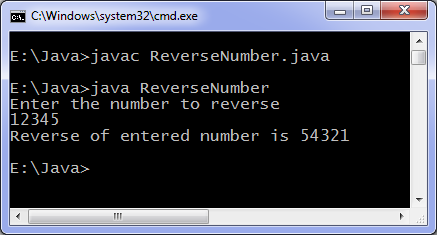# Java program to reverse number

Levels of difficulty: / perform operation:

This program prints reverse of a number i.e. if the input is 951 then output will be 159.

## This java program

```import java.util.Scanner;
class ReverseNumber {
public static void main(String args[]) {
int n, reverse = 0;
System.out.println("Enter the number to reverse");
Scanner in = new Scanner(System.in);
n = in.nextInt();
while( n != 0 ) {
reverse = reverse * 10;
reverse = reverse + n%10;
n = n/10;
}
System.out.println("Reverse of entered number is "+reverse);
}
}
```

### OutputYou can also reverse or invert a number using recursion. You can use this code to check if a number is palindrome or not, if the reverse of an integer is equal to integer then it’s a palindrome number else not.